# Atomic Structure

GCSEAQACombined Science FoundationCombined Science HigherPhysics FoundationPhysics Higher

## Atomic Structure

Atoms are very small building blocks of matter. For a long time, scientists thought that they were the smallest particle but now we know that they are made up of protons, neutrons and electrons

## The Atom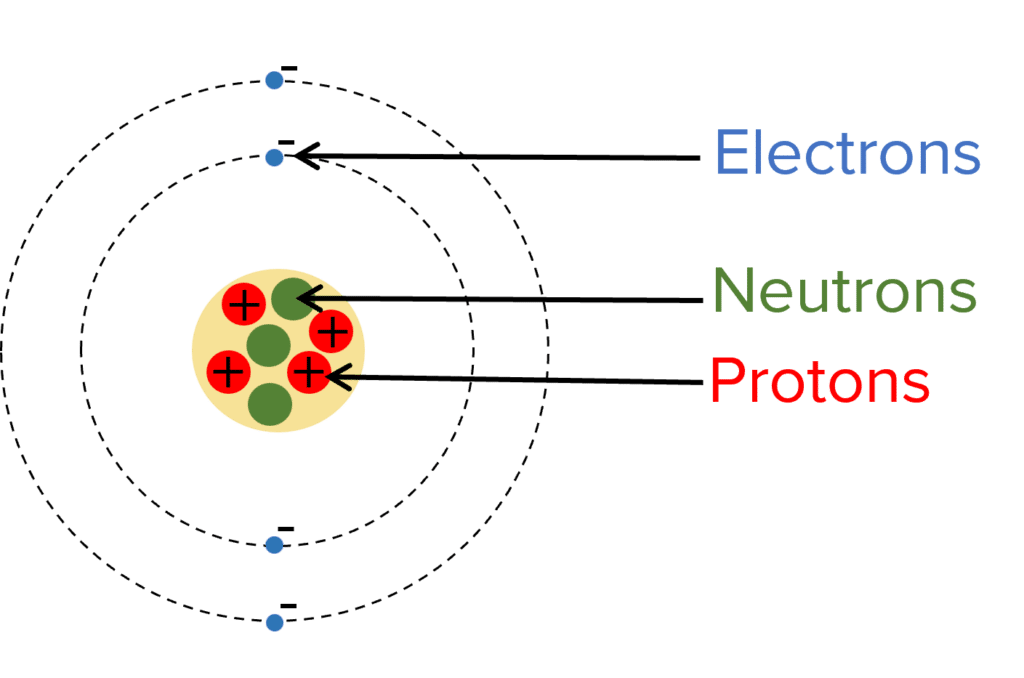Atoms are very small – they have a radius of approximately $\bold{1\times10^{-10}\text{ m}}$. This is $10 \text{ billionths}$ of a metre!

The atom contains a positively charged nucleus at the centre and negatively charged electrons surrounding the nucleus.

The nucleus contains protons, which have positive charge as well as neutrons which are neutral (they have no charge).  The nucleus is very small and does not take up much space. It is more than $10000$ times smaller than the atom however most of the mass of the atom is contained within the nucleus.

Electrons are even smaller than protons and neutrons. They are arranged at multiple distances from the atoms, called energy levels. If they absorb electromagnetic radiation, electrons may go up energy levels and if they release electromagnetic radiation they may go down energy levels.

The number of electrons is equal to the number of protons. As a result, the positive and negative charges cancel and the atom is neutral (no charge).

GCSECombined Science FoundationCombined Science HigherPhysics FoundationPhysics HigherAQA

## Mass Number, Atomic Number and Isotopes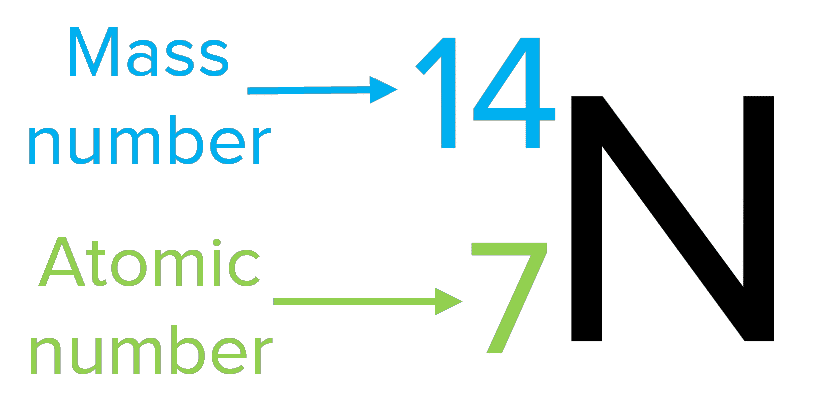All atoms of the same element have the same number of protons. The number of protons is called the atomic number

In a normal atom, the number of electrons is equal to the number of protons and so the atomic number also tells us how many electrons are in the atom.

The mass number is the number of neutrons plus the number of protons. Atoms of the same element may have different numbers of neutrons. Atoms of the same element with different numbers of neutrons are called isotopes. So different isotopes have different mass numbers but the same proton number.

If we know the number mass number and the atomic number, we can calculate the number of neutrons in the atom using:

$\textcolor{f21cc2}{\text{number of neutrons}=\text{mass number}-\text{atomic number}}$

Atoms may also lose or gain electrons. This process is called ionisation and the atoms become ions. These ions are positively charged if they have lost electrons or negatively charged if  they have gained electrons.

GCSECombined Science FoundationCombined Science HigherPhysics FoundationPhysics HigherAQA

## Development of the Model of The Atom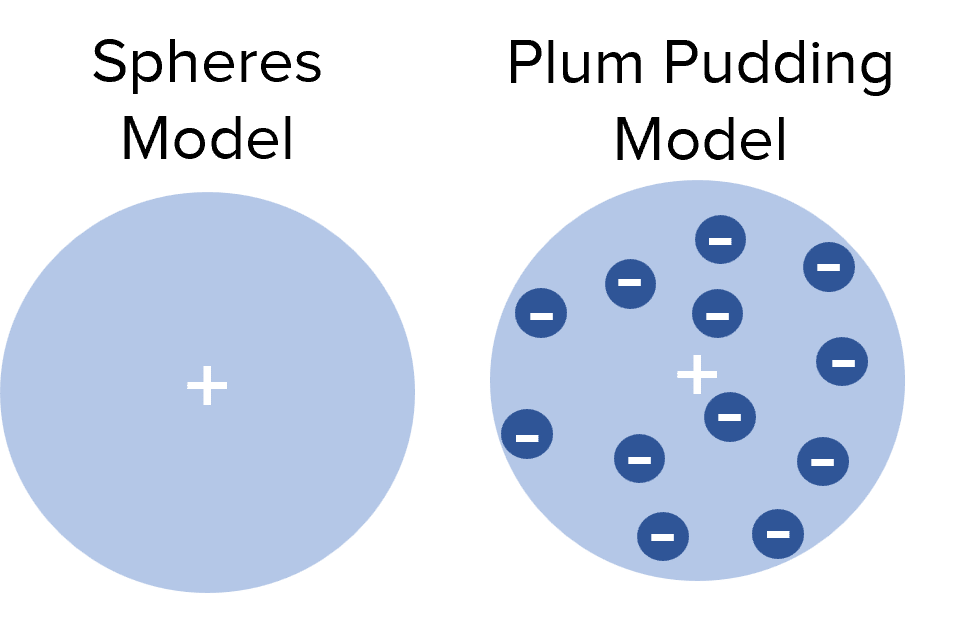In 1803, John Dalton came up with the spherical model of the atom. This model suggests that atoms are tiny spheres that couldn’t be broken into smaller parts.

This spherical model was the accepted scientific opinion until 1909, when JJ Thomson discovered the electron. This lead to the development of the “plum pudding model” where electrons were thought to be distributed randomly in a positively charged sphere, like fruit in a plum pudding.

Then, in 1909, Ernest Rutherford conducted an experiment where he fired positively charged alpha particles at a thin gold foil. He found that most alpha particles went straight through the foil but a small percentage were scattered in different directions. This suggested that the majority of the gold foil must be empty space but there must be small positive charges to reflect the alpha particles.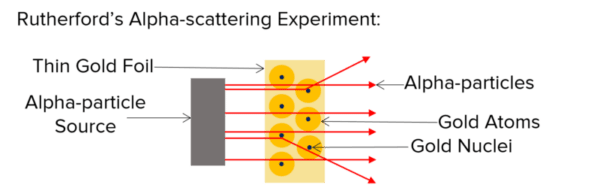From this experiment  Rutherford concluded that:

1. The mass of an atom must be concentrated in a very small and dense central nucleus.
2. The nucleus is positively charged.

These conclusions led to the nuclear model of the atom that we use today. It is possible that in the future, new evidence may be found to change the nuclear model of the atom.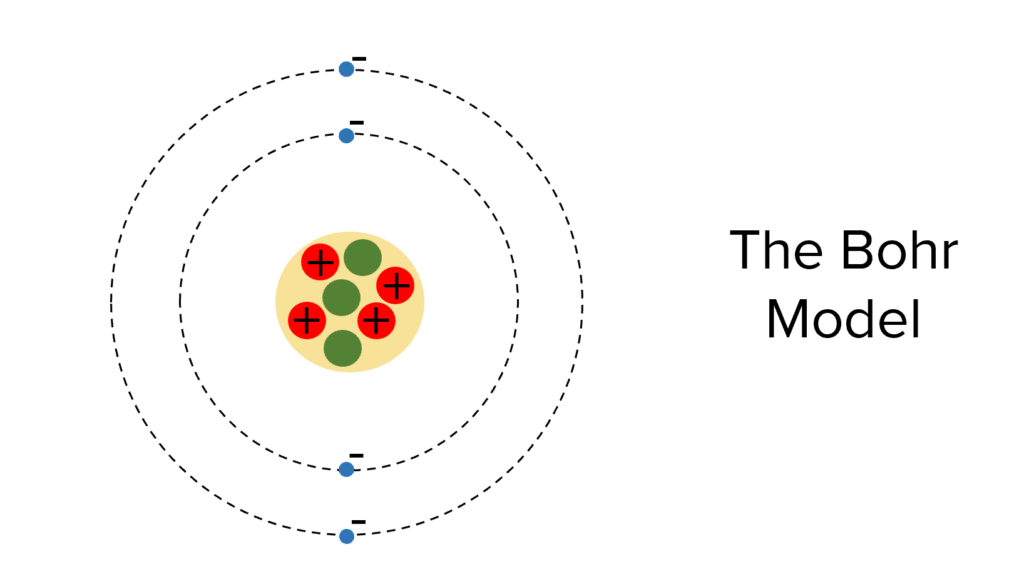Niels Bohr developed the nuclear model of the atom into the model that we use today – the Bohr model. This model has a central nucleus containing protons and neutrons with electron surrounding the nucleus in ‘orbits‘ of specific energies.

James Chadwick found evidence that the nucleus contains neutrons (as well as protons).

GCSECombined Science FoundationCombined Science HigherPhysics FoundationPhysics HigherAQA

## Atomic Structure Example Questions

A: Electron

B: Neutron

C: ProtonGold Standard Education

$\text{atomic number} = \text{number of protons} = \bold{8} \\ \text{mass number} = \text{number of protons} + \text{number of neutrons} = 8+8 = \bold{16}$Gold Standard Education

Atoms of the same element with different number of neutrons/different mass numbersGold Standard Education

1. The mass of an atom must be concentrated in a very small and dense central nucleus.
2. The nucleus is positively charged.Gold Standard EducationProduct